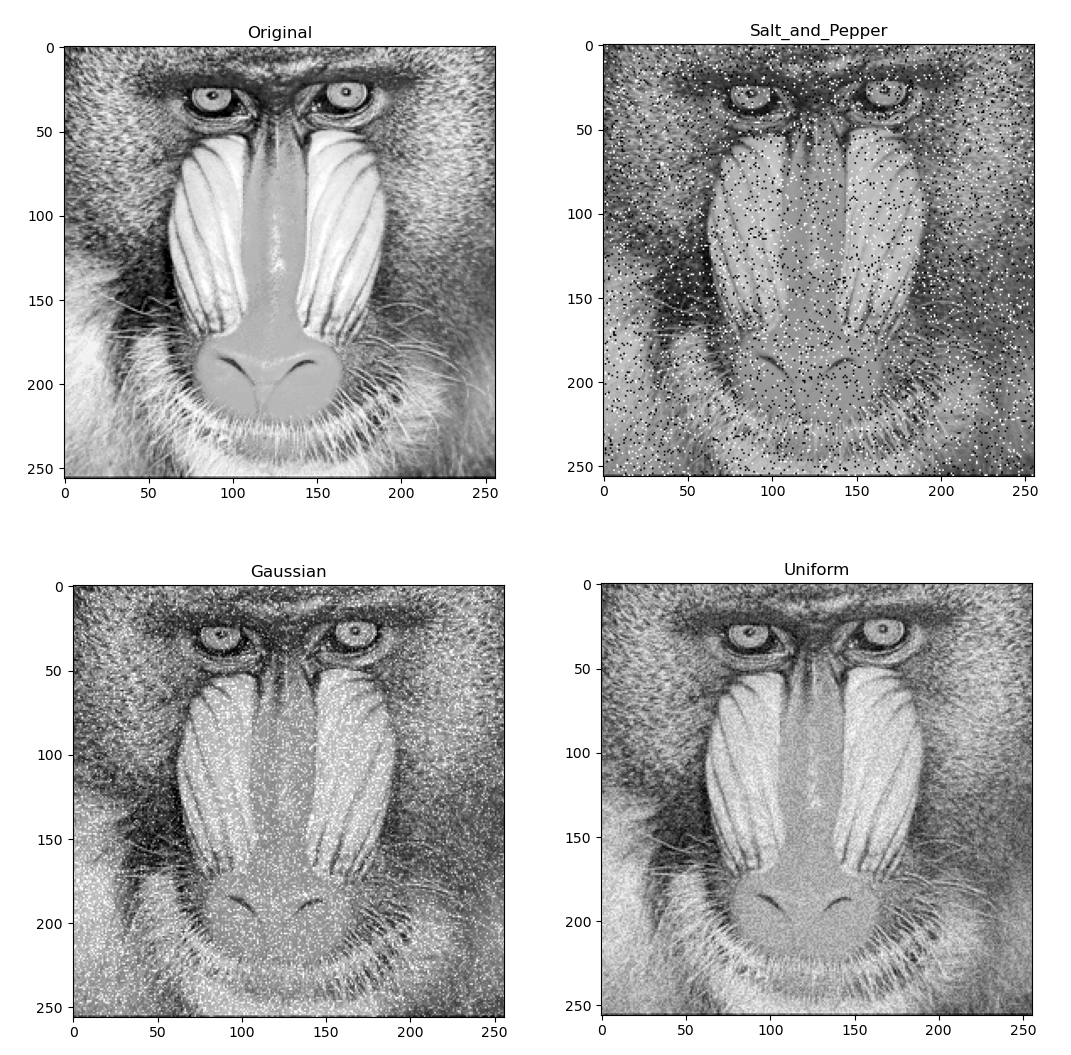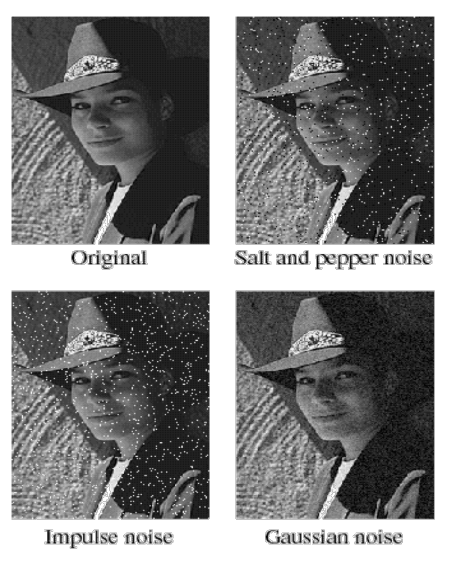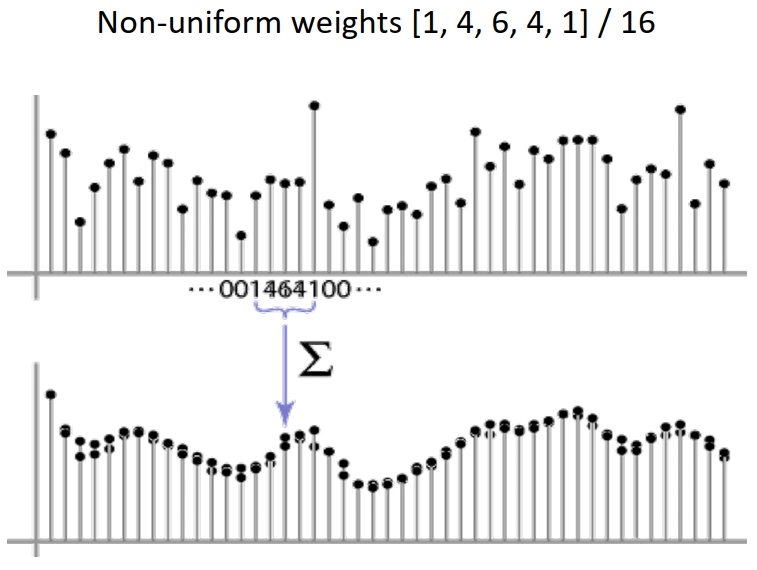# Noise and Filtering in Vision

Noise, denoising, and filtering are very primary concepts in image processing. As machine learning got ubiquitous as a new programming paradigm, people need to know the basics of vision.

The noise is any unwanted added to the data. For example, the following figure shows the concept of a clean signal and a noisy one.

# Noise

Noise is any unwanted added value to the actual data. For example, any unwanted changes are made to the pixels’ values for images. We have three common types of noise in vision:

1. Salt and pepper noise: random occurrences of white and black pixels
2. Impulse noise: Random occurrences of white pixels
3. Gaussian (additive) noise: variations in intensity drawn from a Gaussian normal distribution
4. Uniform noise: A constant value is added to all pixels of an image

In the following pictures, the noises are shown, as discussed earlier.Salt and pepper, Gaussian, Uniform noisesSalt and pepper, Impulse, and Gaussian noises

Gaussian additive noise can be considered as follows:

# Denoising

Unweighted Averaging. Simplest and the first attempt is replacing each pixel with an average of all the values in its neighborhood. The two assumptions in adopting this approach are:

1. Pixels in a neighborhood area resemble each other (entropy of pixels is low), and all pixels in the neighborhood have the same importance
2. Noise is scattered in an independent way of each other (e.g., they are gathered in a neighborhood area)

Weighted Averaging. In this approach, some pixels are considered more important than others. The unweighted average method is like all pixels in a defined neighborhood have weights equal to one.

However, when we give more importance to the pixels closer to the central pixel, we get more smooth results.Weighted average denoising results in smoother output

The calculation is accomplished as the following figure shows. A moving window with weights moves over the image and changes each pixel’s value. The following example shows an unweighted averaging with a 9-pixel window.

## Cross-Correlation Filtering

Each pixel is replaced with a linear combination of its neighbors in this filtering. Usually, the kernel sums to 1.

In OpenCV, a function is used for this operation named filter2D. Consider the following example that moves an image n pixel to the right by building an appropriately-sized kernel.

Blurring kernels or edge detection kernels can be used instead of the shifter kernel in the example above.

## Gaussian Filtering

In this filtering, the nearest neighboring pixels most influence the output.

Choosing the correct value for the mean and variance of the Gaussian filter is crucial for getting acceptable results. Large kernels (over more pixels) may deactivate the denoising effect in some scenarios. However, other strategies would be helpful. The following figure shows that filtering with the Gaussian kernel is smoother than a mean filter.

## Convolution

Because it is a concept that computer science people deal with a lot, I decided to write about it in another post as follows in a more profound and detailed way.

In Convolution, the kernel is flipped on the x and y axes (first one, then the other). The formula shows it. Then, we do what we were doing in correlation filtering. The flipping is because of that minus sign in the convolution formula that the moving kernel has in the back of its independent variable.

Note that we are in discrete numbers realms in images, so we have sums instead of integrals.

The gradient of an image at each pixel shows the direction of rapid change.

The following example shows what it shows:

The direction is given by

The gradient magnitude gives the edge strength.

The following figure shows the image of an eye’s point gradients.

The following figure shows how Convolution can help find an increase in the values of a signal after a specific data point. Also, how helpful is the derivative theorem of Convolution.

# Template Matching

This task aims to find a template or kernel (like an eye) in a bigger image. The main challenge is determining a good similarity or distance between two patches.

The possible measures can be:

1. Correlation
2. Zero-Mean Correlation
3. Sum Square Difference (SSD)
4. Normalized Cross Correlation

Consider the following example that kernel is an eye: